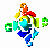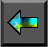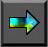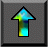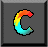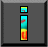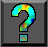Next: Chapter Notes Up: 7 High Performance Fortran Previous: 7.9 Summary

# Exercises

1. Write an HPF program to multiply two matrices A and B of size N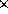N . (Do not use the MATMUL intrinsic!) Estimate the communication costs associated with this program if A and B are distributed blockwise in a single dimension or blockwise in two dimensions.

2. Compare the performance of your matrix multiplication program with that of the MATMUL intrinsic. Explain any differences.

3.   Complete Program 7.2 and study its performance as a function of N and P on one or more networked or parallel computers. Modify the program to use a two-dimensional data decomposition, and repeat these performance experiments. Use performance models to interpret your results.

4. Compare the performance of the programs developed in Exercise 3 with equivalent CC++ , FM, or MPI programs. Account for any differences.

5. Complete Program 7.3 and study its performance on one or more parallel computers as a function of problem size N and number of processors P . Compare with the performance obtained by a CC++ , FM, or MPI implementation of this algorithm, as described in Section 1.4.2. Explain any performance differences.

6.   Develop an HPF implementation of the symmetric pairwise interactions algorithm of Section 1.4.2. Compare its performance with an equivalent CC++ , Fortran M, or MPI program. Explain any differences.

7. Learn about the data-parallel languages Data-parallel C and pC++ , and use one of these languages to implement the finite-difference and pairwise interactions programs presented in this chapter.

8. Develop a performance model for the HPF Gaussian elimination program of Section 7.8, assuming a one-dimensional cyclic decomposition of the array A. Compare your model with observed execution times on a parallel computer. Account for any differences that you see.

9. Develop a performance model for the HPF Gaussian elimination program of Section 7.8, assuming a two-dimensional cyclic decomposition of the array A. Is it more efficient to maintain one or multiple copies of the one-dimensional arrays Row and X? Explain.

10. Study the performance of the HPF global operations for different data sizes and numbers of processors. What can you infer from your results about the algorithms used to implement these operations?

11. Develop an HPF implementation of the convolution algorithm described in Section 4.4.Next: Chapter Notes Up: 7 High Performance Fortran Previous: 7.9 Summary